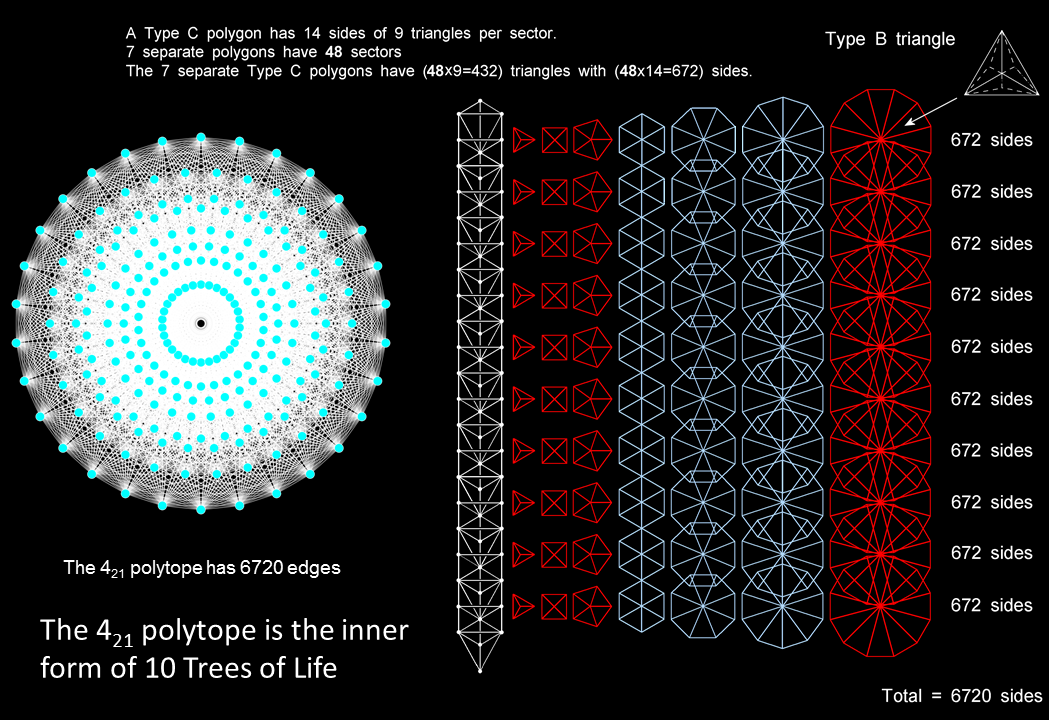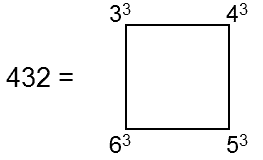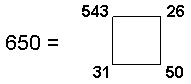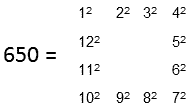Home

 << Previous    1...   20  21  22  23      Next >>

24. The 421 polytope as the inner form of 10 Trees of LifeThe 6720 sides of the 4320 triangles making up the 70 Type C polygons in half of the inner form of 10 Trees of Life correspond to the 6720 edges of the 421 polytope. The 240 corners of these triangles correspond to its 240 vertices. Each half of the inner form of 10 Trees of Life represents a 421 polytope that maps the symmetries of E8. The complete form represents E8×E8. (Click here to view and magnify a PDF version of the 421 polytope)

We found in #21 that 6720 yods other than corners of sectors surround the centres of the 48 sectors of the seven separate polygons of the inner Tree of Life when they are 2nd-order tetractyses. We shall now show that the 70 separate Type C polygons with Type B triangles as their sectors generated by 10 overlapping Trees of Life contain 6720 sides that match the 6720 edges of the 421 polytope.

The Type B triangle is composed of seven corners & 15 sides of nine triangles, i.e., 31 geometrical elements, where 31 is the number value of EL, the Godname of Chesed. The number of sides of the 9n triangles in a Type C n-gon with Type B triangles as its n sectors = 14n, as is the number of its corners & triangles surrounding its centre. Hence, the number of sides of the (9×48=432) triangles in the seven separate polygons of the inner Tree of Life = 14×48 = 672. If we now consider 10 overlapping Trees of Life, the 70 separate polygons that make up their inner form have (432×10=4320) triangles with (672×10=6720) sides. This is the number of edges in the 421 polytope. Moreover, the nine triangles in each sector have five corners other than the centre of the polygon, so that the 432 triangles in the 48 sectors have 240 corners other than the seven centres of the polygons. The 4320 triangles in the 70 separate Type C polygons have 2400 corners, where

2400 = 492 − 1 = 3 + 5 + 7 + ... + 97

is the sum of the first 48 integers after 1 ending with 97, the number value of Haniel, the Archangel of Netzach. Compare this with the fact that the 421 polytope has 240 vertices that define the 240 roots of E8 because their position vectors are its non-zero root vectors in 8-dimensional space. They in turn define 240 gauge charges that are coupled to 240 10-dimensional gauge fields with 2400 space-time components. It is as though the 240 corners symbolise the 240 gauge fields, the 2400 components of which correspond to the 2400 corners of the 70 polygons that make up the 10 Trees of Life mapping the 10 dimensions of superstring space-time. This is remarkable evidence of the 421 polytope as the inner form of 10 Trees of Life. Notice that the number of corners & triangles = 240 + 432 = 672, which is the same as the number of their sides. It means that the number of geometrical elements surrounding the centres of the seven Type C polygons = 2×672. The seven separate polygons can be divided in two ways into two sets with 24 corners:

1. triangle-square-pentagon-dodecagon & hexagon-octagon-decagon
2. triangle-pentagon-hexagon-decagon & square-octagon-dodecagon

(the diagram illustrates the former division). Each set of three or four polygons contains (9×24=216) triangles with (5×24=120) corners and (14×24=336) sides, i.e., 672 geometrical elements. Each set of 30 or 40 polygons generated by 10 Trees of Life has 2160 triangles with 1200 corners and 3360 sides (6720 geometrical elements). They correspond to the 3360 edges in each half of the 421 polytope. Article 64 analyses the 24:24 division in the seven polygons of the inner Tree of Life. Its two halves correspond to the two halves of the 421 polytope.

There are 432 triangles in each set of seven separate Type C polygons, whereThe four separate, red polygons with 24 corners have (63=216) triangles, whilst the remaining three separate, light blue polygons with 24 corners have (33+43+53=216) triangles.

To complete the discussion, let us now determine the numbers of corners, sides & triangles making up the seven enfolded Type C polygons. The seven enfolded, basic polygons have 47 sectors with 41 corners and 88 sides. Inside a Type B triangle are four corners & 12 sides of nine triangles, i.e., 25 geometrical elements. Inside the 47 Type B sectors are (4×47=188) corners & (12×47=564) sides of (9×47=423) triangles. The seven enfolded, Type C polygons have (41+188=229) corners & (88+564=652) sides of 423 triangles, i.e., 1304 geometrical elements. The (7+7) enfolded, Type C polygons have 456 corners & 1303 sides of 846 triangles, i.e., 2605 geometrical elements.

• 229 is the 50th prime number. This is how ELOHIM, the Godname of Binah with number value 50, prescribes the number of points defining the shapes of the seven enfolded polygons when they are constructed from triangles. The number of corners outside the root edge = 227. This is the 49th prime number, showing how EL CHAI, the Godname of Yesod with number value 49, prescribes the part of the inner Tree of Life outside its root edge.
• Of the 652 sides of the 432 triangles making up the seven enfolded, Type C polygons, two sides are shared with the outer Tree of Life. They are the Chokmah-Chesed Path and the Chesed-Netzach Path, which coincide with the two internal, vertical sides of sectors of the hexagon. 650 (=65×10) sides are unshared and therefore intrinsic to the inner Tree of Life. ADONAI, the Godname of Malkuth with number value 65, prescribes its shape. The Decad determines the number 650 because 65 is the sum of the first 10 integers after 1:
 2 3 4 65 = 5 6 7 8 9 10   11
• 650 is also the sum of the numbers of the Godnames of the first four Sephiroth:It is also the sum of the first twelve squares:• The two separate halves of the complete inner Tree of Life made of (7+7) enfolded Type C polygons have 1300 sides of 846 triangles that are unshared with the outer Tree of Life, where

1300 = 15 + 25 + 35 + 45.

This is the number of yods other than corners of polygons in the (7+7) enfolded Type B polygons because the latter have 1370 yods, of which 70 yods are polygonal corners.

•

 << Previous    1...   20  21  22  23      Next >>

Home